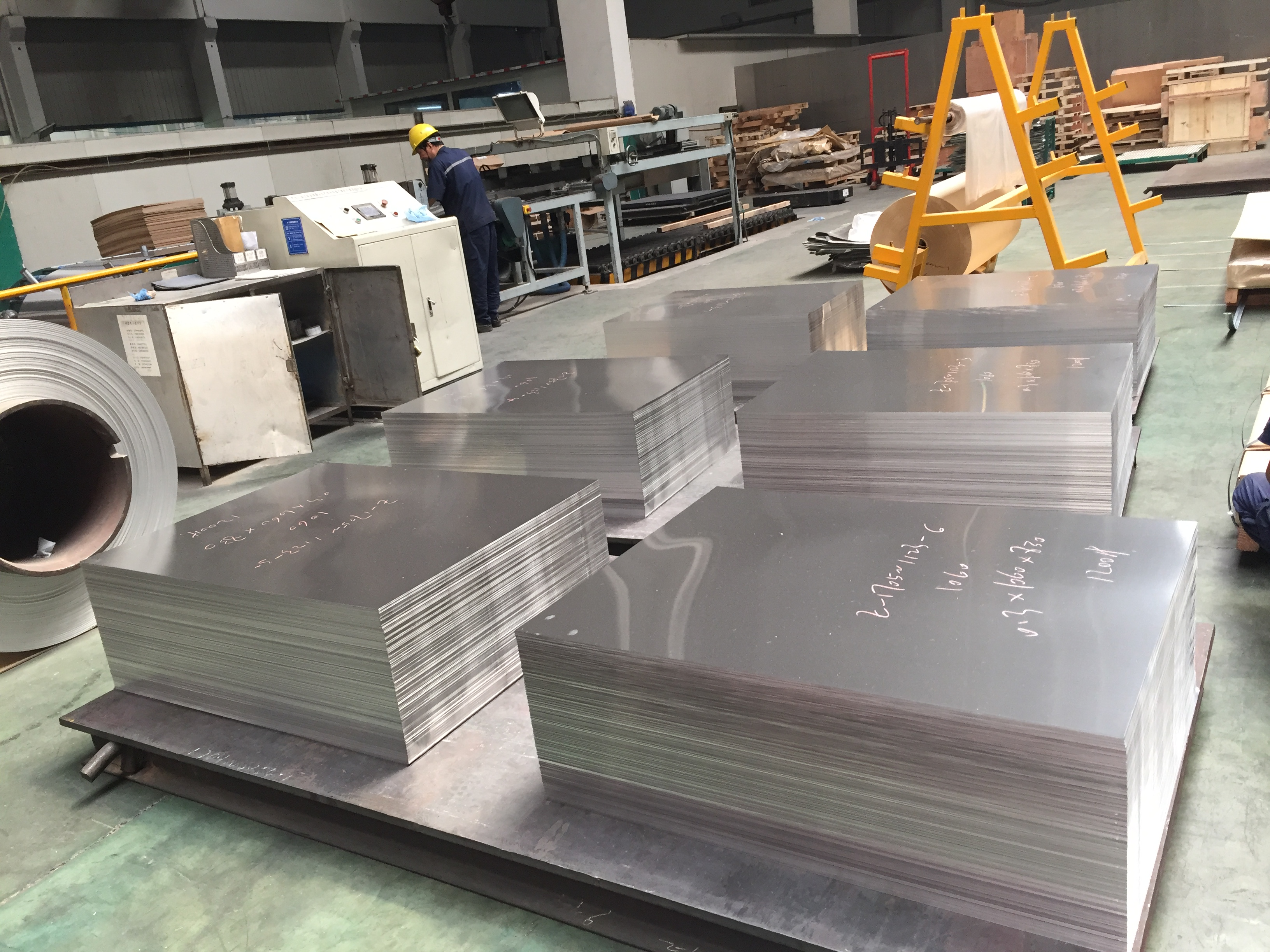# The Calculation of the Weight of the Aluminum Sheet

The aluminum sheet is applied widely, and the weight is required to be calculated in many occasions, so you may ask how can we calculate the weight of the aluminum sheet quickly, the answer will be given as follows: cause the density of the aluminum sheet is 2,71, and the weight of it can be calculated in the formula: the weight=2.71×length(m) ×width(m), for example, there is an aluminum sheet, of which the length is 1 m, the width is 2 m and the thickness is the 3 m, then the calculating method can be the 1×2×3×2.71=16.26, so we can finally get the weight of this aluminum sheet is 16.26 kg. Of course, it is the general way of calculating, and it the purity of the aluminum sheet is different, and the value of the density is different, the result will be different, so you had better make sure the value of the density before calculating.the Aluminum Sheet

The Haomei Industrial Co., Ltd is the professional aluminum sheet manufacturing company in China, we supply all kinds of aluminum sheet, which will satisfy your different demand, if want to know more information about the aluminum sheet, you can visit the website of the Haomei, we share many articles on it, and there are also the products introduction on it, and you can consult the professional salesman and they will answer your question patiently.